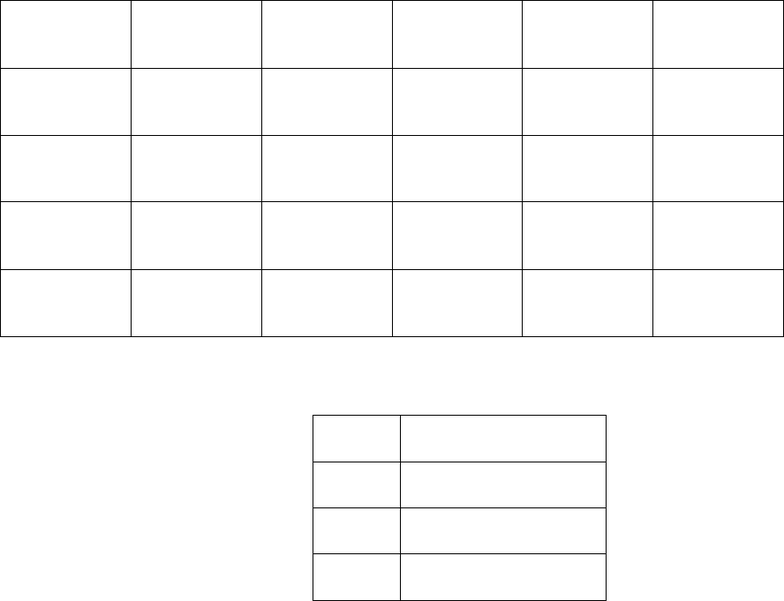Class Notes (1,100,000)
CA (620,000)
Carleton (20,000)
ECON (300)
ax (1)
Lecture 1

# ECON 2102 Lecture Notes - Lecture 1: Quantitative Easing, Household Debt, Gdp Deflator

Department
Economics
Course Code
ECON 2102
Professor
ax
Lecture
1

This preview shows pages 1-3. to view the full 10 pages of the document.ECON 311 - Intermediate Macroeconomics (Professor Gordon)
First Midterm Examination: Fall 2011
Circle the TA session you attend: Ofer 9AM 4PM
Nuri 4PM
Juan 9AM
INSTRUCTIONS:
1. The exam lasts 1 hour.
2. The exam is worth 60 points in total: 30 points for the multiple choice questions and 30 points for the two analytical
problems.
3. Write your answers for part A (the multiple choice section) in the blanks below. You won’t get credit for circled
answers in the multiple choice section.
4. Place all of your answers for part B in the space provided.
5. You must show your work for part B questions. There is no need to explain your answers for the multiple choice
questions.
6. Good luck!
PART A: Multiple Choice Problems
Answer multiple choice questions in the space provided below.
1) 2) 3) 4) 5) 6)
7) 8) 9) 10) 11) 12)
13) 14) 15) 16) 17) 18)
19) 20) 21) 22) 23) 24)
25) 26) 27) 28) 29) 30)
MC
Q1
Q2
Total

Only pages 1-3 are available for preview. Some parts have been intentionally blurred.

PART A: Multiple Choice Problems
1) The inflation rate is the
A) measure used to calculate the price level.
B) measure used to calculate the cost of borrowing money.
C) percentage increase in the average level of prices.
D) percentage increase in the average level of wages.
2) The multiplier measures the
A) number of steps it takes to move from one equilibrium to another.
B) rise in saving resulting from a rise in income.
C) marginal propensity to invest.
D) rise in equilibrium GDP resulting from a one dollar rise in planned autonomous expenditures.
3) "Real money balances" refers to
A) the currency part of the total money supply.
B) the money supply divided by the price level.
C) the money supply times one minus the interest rate.
D) the non-interest-earning part of the money supply.
4) The average output produced per worker is one way of measuring
A) inflation.
B) the interest rate.
C) employment.
D) productivity.
5) Which of the following would shift the LM curve?
A) an increase in the tax rate
B) an increase in the real money supply
C) a reduction in business confidence
D) All of these.

Only pages 1-3 are available for preview. Some parts have been intentionally blurred.

6) The cars produced by Toyota (a Japanese company) in its factory in Kentucky ________ in Japanese GDP.
Distributed profits to Toyota’s shareholders _______ in Japanese GNP (assume that Toyota’s owners are all
Japanese).
A) count, count
B) count, do not count
C) do not count, count
D) do not count, do not count
7) If a the government of Country Z is running a budget deficit and net exports are zero, then
A) investment is greater than saving.
B) investment and saving are equal.
C) saving is greater than investment.
D) none of the above.
8) If real GDP for a given year is \$2400 billion and nominal GDP is \$2400 billion,
A) this year is the base year for the GDP deflator.
B) the GDP deflator for this year is 1.25.
C) the GDP deflator for this year is 0.8.
D) the GDP deflator for this year is 1.10.
9) Help in financing our federal budget deficit comes from a ________ amount of net exports, which is called
________.
A) negative, net foreign investment.
B) negative, foreign borrowing.
C) positive, net foreign investment.
D) positive, foreign borrowing.
10) Which of the following is not a reason why an increase in stock market wealth reduces the personal saving
rate
A) Autonomous consumption depends positively on real net worth
B) Autonomous consumption depends negatively on the interest rate
C) Higher stock prices generate capital gains
D) Saving equals disposable income minus consumption